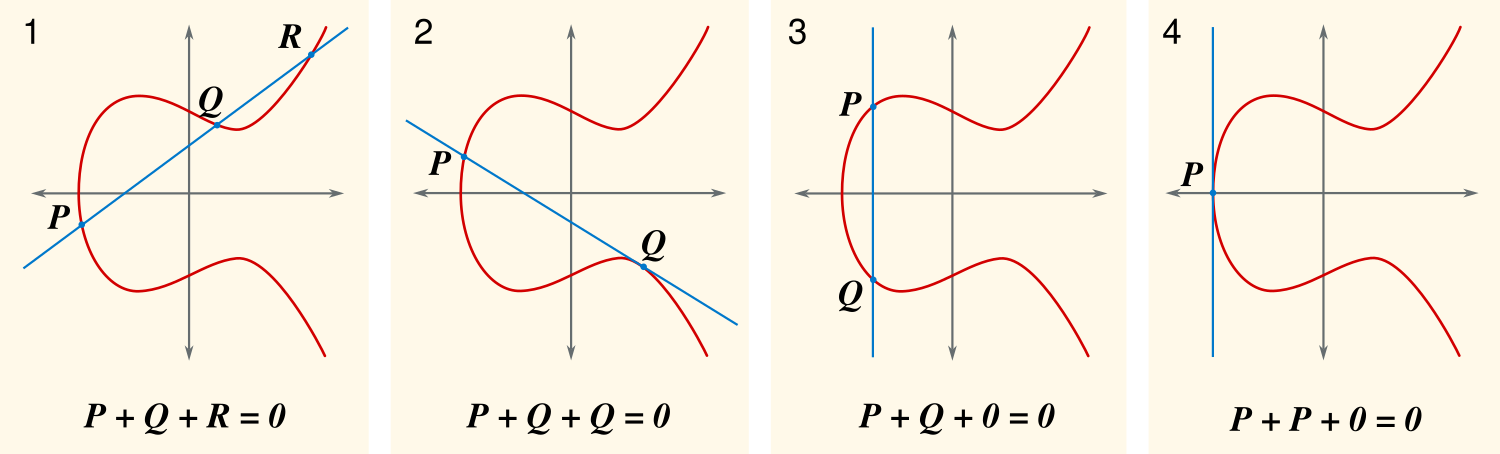Sat Jun 02 2018

# Public Key Cryptography using Elliptic Curves

Trapdoor functions or one-way functions are essential for the working of public key cryptography or asymmetric cryptography where computing the reverse of the function is not feasible by modern day computers. Multiplication of two primes is easy but factoring a product of two primes is difficult, and this forms the basis of RSA cryptography, the widely used public key cryptography system. If you do not know what RSA is, I suggest reading this. In this post, I talk about another asymmetric cryptography system known as Elliptic Curve Cryptography.

## Elliptic Curve Cryptography

Elliptic curves are planar curves defined by the following equation.

where and are constant and to avoid singular points. The elliptic curves are symmetric about the x-axis.

Geometrically they look like this:Source: By Tos [Public domain], from Wikimedia Commons

To use elliptic curves for cryptography, a random large prime, (or a perfect power of ) is chosen, and all the points on the curve are the points satisfying equation where each operation is under modulo . The elliptic curve over the finite field of integers modulo would look like this:Source: By trshaffer [CC0], from Wikimedia Commons

Now we define addition and scalar multiplication among the points on the plane.

Consider two points, and on the curve, now draw a line joining and . This line intersects the curve at another point . Let the reflection of the point about x-axis be . Since elliptic curves are symmetric about the x-axis, is also on the curve and is denoted .

Under modulo arithmetic, the XY plane is bounded. The line joining and once when crosses the bounded plane on one side wraps around and comes out from the other side of the plane at the opposite point with slope same as the original slope and continue further until it intersects at some point on the curve.

Now, consider and its reflection of about the x-axis as . The line joining and never intersect the curve. We can think of it as the line meeting the curve at infinity(), i.e. .

We call as the inverse of and as the identity under this specially defined addition operation. is also written as .

### Scalar Multiplication

Lets first define . For this, we consider the tangent at and find the reflection about the x-axis of the point at which the tangent intersects the curve. This point is defined as . Inductively, we can define (scalar multiplication).

Let . Since all these points are over the finite field of integers modulo , it turns out that computing from and is easy, but it is not feasible to compute from and . The reason for infeasibility is the difficulty of computing discrete logarithm in this finite field. This scalar multiplication is the one-way function that forms the basis of the elliptic curve cryptography.

## Encryption and Decryption

There are many proposed algorithms for encryption/decryption using elliptic curves. The one I describe here is analogous to ElGamal public key encryption algorithm.

Read more: Handouts on ElGamal Algorithm, MCS 425, Spring 2008, Dept. of Mathematics, Statistics and Computer Science, UIC

Consider that Alice wants to send a message to Bob. Both Alice and Bob decide on the elliptic curve equation, and a point on the curve called as the generator. The recommended equation and generator pair is given by Standards of Efficient Cryptography. Bob chooses a random number and calculates . The integer forms the private key and forms the public key.

Alice chooses a random integer (each time Alica has to choose different ) and calculates the cypher text as follows:

Here is the public key of Bob.

Bob receives the cypher text and decrypts the message as follows:

## Conclusion

ECC with a private key of size 256 bits offers the same security as RSA used with 3072 bits size key1. Hence ECC is more used when compared to RSA for mobile devices where the size of the key matters. The operation of encryption and decryption is symmetric in case of RSA hence the same can be used for Digital Signatures, but it is not the same in ECC, and hence a different algorithm is used for signing2.